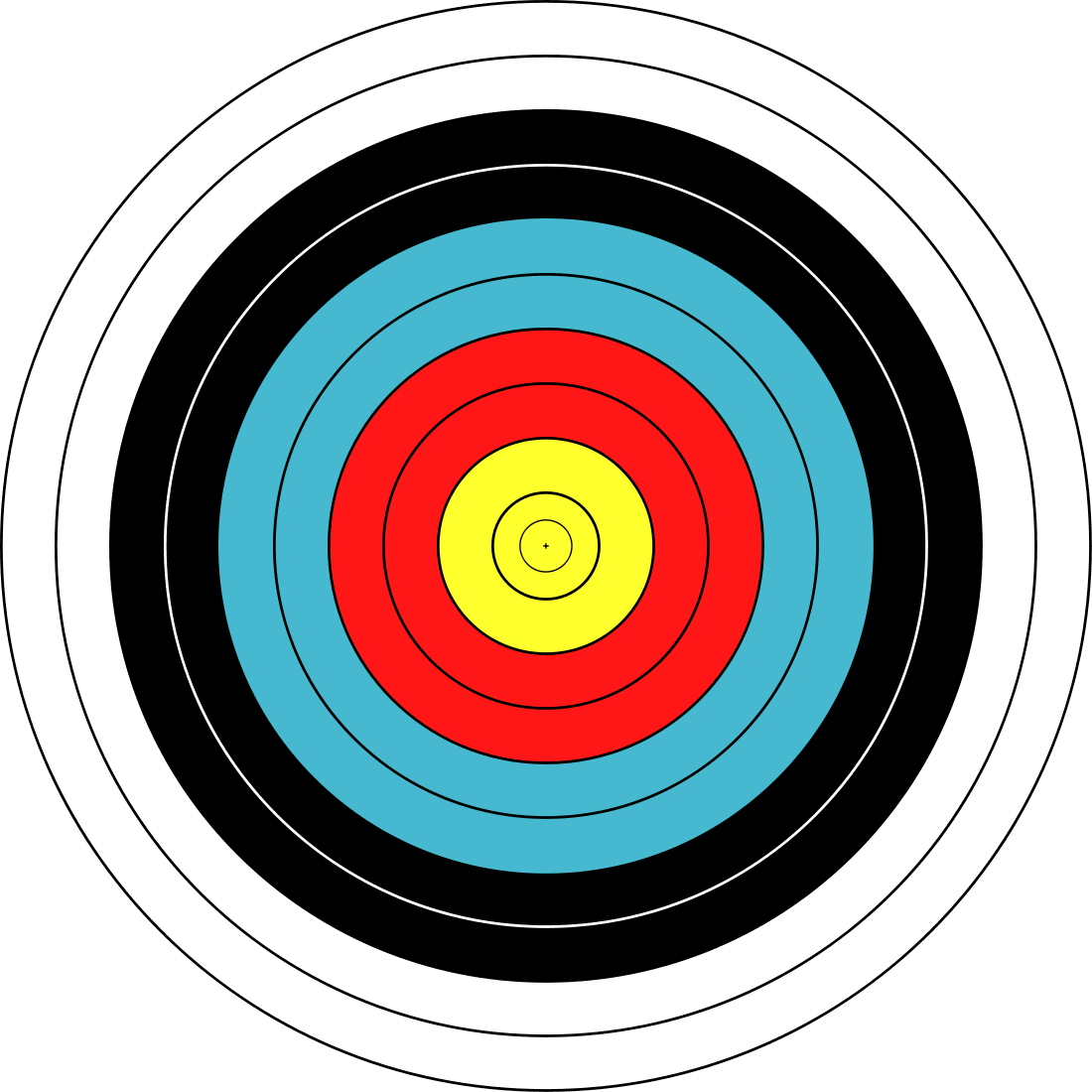# Quiz 3a#

## Question 1#

What Python operator takes two strings (e.g., `"sun"` and `"rise"`) and forms the combination of these two strings, one followed by the other (e.g., `"sunrise"`)?

`+`
`*`
`append`
`concat`
`concatenate`\

`+`\

## Question 2#

What does the draw handler parameter represent?

If you’ve forgotten, refer to the documentation .

The object to be drawn
Nothing — it doesn’t have a parameter
The canvas
The frame
The location of the object to be drawn \

The canvas \

## Question 3#

What happens if you draw text outside the canvas coordinates?

Try it in CodeSkulptor .

The text appears in the frame, but some or all of the text is shown outside the canvas area.
Some or none of the text is shown. Conceptually, the text is drawn at whatever coordinates are given, but only whatever text fits within the canvas coordinates is shown.
Causes an error
The text coordinates are implicitly “wrapped” around using modular arithmetic, so that the text appears on the canvas \

Some or none of the text is shown. Conceptually, the text is drawn at whatever coordinates are given, but only whatever text fits within the canvas coordinates is shown. \

## Question 4#

Assume we have a canvas that is 200 pixels wide and 300 pixels high. We want to draw a green line between the upper left corner of the canvas and the lower right corner of the canvas. Which of the following calls will accomplish this?

Try the code in CodeSkulptor .

```  canvas.draw_line((0, 0), (300, 200), 10, "Green")

```

\

```  canvas.draw_line((200, 0), (0, 300), 10, "Green")

```

\

```  canvas.draw_line((200, 300), (0, 0), 10, "Green")

```

\

```  canvas.draw_line((200, 300), (0, 0), 10, "Green")

```

## Question 5#

Consider the following function definition.

```def date(month, day):
"""Given numbers month and day, returns a string of the form '2/12',
with the month followed by the day."""
return month   "/"   day

print date(2, 12)
```

This definition leads to an error. To fix it, what Python expression should replace the question marks below?

```def date(month, day):
"""Given numbers month and day, returns a string of the form '2/12',
with the month followed by the day."""
return #???

print date(2, 12)
```

`str(month) + "/" + str(day)`
`string(month) + "/" + string(day)`
`str(month + "/" + day)`
`str(month / day)`
`string(month + "/" + day)`
`string(month / day)`\

`str(month) + "/" + str(day)`\

Also possible: `print(month, '/', day)`

## Question 6#

Assume we have a variable `word` that contains a string, such as `"Mississippi"` or `"indivisible"`. We would like to determine how many `"i"`’s are in the string `word`. What code should replace the question marks in the following function definition?

```def number_of_i(word):
"""Returns the number of lower-case i's in the word."""
return ???
```

There is a built-in function or method that will do this. Look in the CodeSkulptor documentation for the appropriate one.

`count(word, "i")`
`word.count("i")`
`count("i", word)`
`"i".count(word)`\

`word.count("i")`\

## Question 7#

Where should your `draw_text`, `draw_line`, and similar drawing calls be?

In the handlers for the control objects that create or change the drawing, or their helper functions
In a draw handler, or a helper function called from it \

In a draw handler, or a helper function called from it \

## Question 8#

Which of the following function calls are valid, i.e., don’t lead to an error?

Read the documentation for these functions, and also try the code in CodeSkulptor.

`float("5 five")`
`int("5.4")`
`float("5.4")`
`int("5")`\

`float("5.4")`
`int("5")`\

## Question 9#

Turn the following description into a CodeSkulptor program, and run it.

1. Create a 300-by-300 canvas.

2. Draw two circles with radius 20 and white lines of width 10. One is centered at (90,200) and one at (210,200).

3. Draw a red line of width 40 from (50,180) to (250,180).

4. Draw two red lines of width 5 from (55,170) to (90,120) and from (90,120) to (130,120).

5. Draw a red line of width 140 from (180,108) to (180,160).

The resulting picture is a simple diagram of what?

A motorcycle
A house
An automobile
A person
A computer \

```try:
import simplegui
except ModuleNotFoundError:
#import SimpleGUICS2Pygame.simpleguics2pygame as simplegui
import simplequi as simplegui

def draw(c):
c.draw_circle([90, 200], 20, 10, 'white')
c.draw_circle([210, 200], 20, 10, 'white')
c.draw_line([50, 180], [250, 180], 60, 'red')
c.draw_line([55, 170], [90, 120], 5, 'red')
c.draw_line([90, 120], [130, 120], 5, 'red')
c.draw_line([180, 108], [180, 160], 140, 'red')

f = simplegui.create_frame('', 300, 300)
f.set_draw_handler(draw)
f.start()
```

an automobile (transporter)

## Question 10#

The following is a diagram of an archery target.{assetid=”C74TXbr4EeW43wpBjgOOlw”}

To draw this in CodeSkulptor, we can put a small yellow circle with a black border on top of a slightly bigger yellow circle with a black border, … on top of a big white circle with a black border. In what order should your code draw these circles?

Largest first
Smallest first \

Largest first \